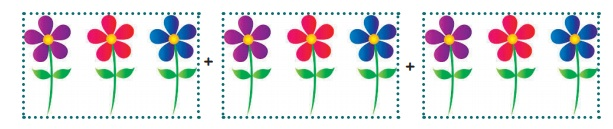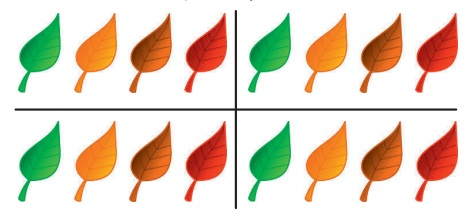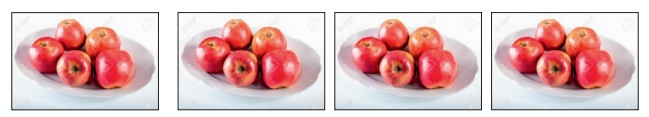Home | | Maths 3rd Std | Repeated addition

## Chapter: 3rd Maths : Term 2 Unit 1 : Numbers

Let us recall the repeated addition we have learnt in lower classes.

Mutiplication of a number with other number can be done in the following ways.

(i) Dot multiplication

(iii) Regrouping

(iv) Standard multiplication algorithm

(v) Lattice multiplication

Let us recall the repeated addition we have learnt in lower classes.

i) Find the total number of flowers.We can find the total number of flowers as follows.

3 + 3 + 3 = 9

3 groups of 3 flowers make 9

3 × 3 = 9

ii) Find the total number of leaves.4 + 4 + 4 + 4 = 16

4 × 4 = 16

iii) How many apples are there in four platesThere are four plates. Each has five apples.

Total number of apples = 5 + 5 + 5 + 5 = 20

5 × 4 = 20

Tags : Numbers | Term 2 Chapter 1 | 3rd Maths , 3rd Maths : Term 2 Unit 1 : Numbers
Study Material, Lecturing Notes, Assignment, Reference, Wiki description explanation, brief detail
3rd Maths : Term 2 Unit 1 : Numbers : Repeated addition | Numbers | Term 2 Chapter 1 | 3rd Maths